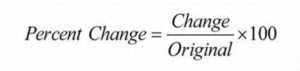# MS Math – Nov 29-Dec 3We hope you had a wonderful Thanksgiving!

6th Grade Math – Mrs. Evans

This week we will continue the study of ratios, rates and unit rates in Chapter 4. Rates and unit rates are very useful mathematical concepts that we use in day to day life, such as when we buy things (\$/packages) or maintain a safe speed in the car (miles/hour).  Take the opportunity to discuss as many of these situations that your family encounters this week!  Here is a video to help your student understand Ratios, Rates and Unit Rates:

Math Grade Math – Mrs. Evans and Mrs. VonFeldt

This week, we will do what we had planned to do last week – start Module 5!    We will learn about finding the percent change in different amounts.  I include this from last week because it did not get covered last week.We will be determining the markup and markdown on quantities using percentages.  Finding markup and markdown requires thinking logically.  Here is a video about percent change to introduce you to this useful concept.

We will use this concept in solving real world problems.  As a family you can call attention to all the places that we use percentages in day to day life; when we shop, eat out, leave a tip, pay taxes, make interest on our savings or pay interest on loans.  It is a great time to talk about these situations with your student!

8th Grade Math – Mrs. Ernest

The students are learning how to apply exponent rules to Scientific Notation this week! We will begin by learning the Scientific Notation format, then we will practice converting large and small numbers into Scientific Notation. Next, we will work backwards- turning a number written in the notation back to a large or small number. Students will learn how to multiply and divide Sci Notation numbers, and if we have time, we will begin to apply all of our learning about exponents to a new concept: Square Roots!

Algebra – Mrs. VonFeldt

This week, we will continue to practice writing and graphing linear equations. Students will learn how to express a line in point-slope form. This form is particularly helpful when you are given a slope and a random point on the line. Then, they will learn how to rewrite that equation into slope-intercept form or standard form. Students should be able to go back and forth between these three forms by rewriting the equation.

© 2022 Assumption Catholic School | 2116 Cornwall Ave, Bellingham, WA 98225
Phone: 360.733.6133 | Fax: 360.647.4372
Email: theoffice@school.assumption.org
Dashboard | Web design and development by Olywebdev.com